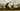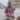# Useful Details About Underscore Keyword in Swift

Posted October 25, 2016Start discussion

Swift is known for its ability to help creating readable and self explanatory code. Such practice is efficient, because writing meaningful code has a big impact on understanding: and as result on productivity.

The language offers useful syntax to improve the readability using argument labels, or to access a tuple or structure component.

For instance the following function returns a tuple that contains the minimum and maximum values from the provided two arguments:

`swift`func getMinMax(a: Int, b: Int) -> (Int, Int) {  if (a < b) {    return (min: a, max: b)  }  return (min: b, max: a)}let (min, max) = getMinMax(a: 15, b: 8)print(min) // => 8print(max) // => 15``

The invocation `getMinMax(a: 15, b: 8)` has `a` and `b` as argument labels. These indicate additional information about the arguments.

Every medal has two sides. There are situations where verbose code becomes redundant. It talks too much than it’s necessary.

Thinking better about the previous example, it is possible that `a` and `b` argument labels do not provide useful information. And suppose you want to access only the minimum from the returned tuple `let (min, max) = getMinMax()`.

For such cases Swift provides the wildcard pattern - a special underscore character `_`.

Let’s use the underscore and transform the previous example:

`swift`func getMinMax(_ a: Int, _ b: Int) -> (Int, Int) {  if (a < b) {    return (min: a, max: b)  }  return (min: b, max: a)}let (min, _) = getMinMax(15, 8)print(min) // => 8``

The function signature is changed to `getMinMax(_ a: Int, _ b: Int)`, where `_` is inserted before the parameter name. This means that argument labels are not necessary on invocation: `getMinMax(15, 8)`.
Also the max component of the tuple can be skipped on extraction: `let (min, _)`.

Let’s study the situations where underscore `_` helps avoiding redundant details.

## 1. Omitting argument labels

Starting version 3.0 Swift requires by default to indicate the argument labels on invocation.
The parameter name `paramName` in `func myFunc(paramName: Type) {...}` automatically create argument label `paramName:` on invocation `myFunc(paramName: valueOfType)`.

Let’s see a simple case:

`swift`func sum(x: Int, y: Int) -> Int {  return x + y}print(sum(x: 10, y: 5)) // => 15``

When invoking `sum` function, you have to indicate the argument labels `x: ` and `y: ` for both arguments: `sum(x: 10, y: 5)`.

As mentioned in the introduction, if you want to omit the argument label, insert an underscore before the parameter name: `func sum(_ x: Int, ...) {...}`.

Let’s modify the previous example:

`swift`func sum(_ x: Int, _ y: Int) -> Int {  return x + y}print(sum(10, 5)) // => 15``

Since the argument label is not necessary, the invocation `sum(10, 5)` looks better. It’s clear that `10` and `5` are the operands in a sum operation. No more details are necessary.

You can use `_` to suppress the argument label every time its indication seems redundant.

## 2. Ignore tuple component

Tuples are simple data structures that are used to couple related values. They are especially useful when returning multiple values from a function.

Let’s create a complex function that executes 4 arithmetic operations:

• Subtraction
• Multiplication
• Division

Since all the operations are related, they are returned in a tuple:

`swift`func calculateOperations(_ first: Int, _ second: Int)                        -> (add: Int, sub: Int, mul: Int, div: Int) {  return (    add: first + second,    sub: first - second,    mul: first * second,    div: first / second  )}let (add, sub, mul, div) = calculateOperations(8, 6)print(add, sub, mul, div) // => 14 2 48 1``

`let (add, sub, mul, div)` extracts the tuple components into variables.

Suppose you need to access only the subtraction and division results: the underscore fits nice it this situation. Replace the unnecessary variables `add` and `mul` with an underscore `_`:

`swift`// ...let (_, sub, _, div) = calculateOperations(8, 6)print(sub, div) // => 2 1``

`let (_, sub, _, div)` contains `_` at first and third positions. This indicates that addition and multiplication results are insignificant.

Alternatively you can retrieve the tuple instance into a variable, and use a selector to access the named tuples components:

`swift`// ...let result = calculateOperations(8, 6)print(result.sub, result.div) // => 2 1print(result.1, result.3)     // => 2 1``

`result.sub` and `result.div` (or `result.1` and `result.3`) selects tuple’s named components `sub` and `div`.
The downside of this approach is the need to keep a variable for tuple. And later access the components using a selector.

## 3. Ignore enumeration associated value

Swift is so kind that besides simple enumeration, you can associate for each enumeration case a value. These are similar to tagged unions data structure from computer science.

Enumeration is a powerful data structure, especially in combination with associated values. However extracting data from such complex structure has some overhead, especially to access the associated values.

For instance let’s enumerate the flight phases of an aircraft. An aircraft can:

• Stay on the ground without movement (`fixed`)
• It can accelerate with a specific speed before take-off (`acceleration (Int)`)
• It can fly with a specific speed and altitude (`flight(Int, Int)`)
• And land with a specific speed (`landing(Int)`)

Let’s code the enumeration:

`swift`enum FlightPhase {  case fixed  case acceleration(Int)  case flight(Int, Int)  case landing(Int)}``

One of the most important characteristic of a flying aircraft is the speed. Let’s add to enumeration a new method `getSpeed()`:

`swift`enum FlightPhase {    case fixed  case acceleration(Int)  case flight(Int, Int)  case landing(Int)  func getSpeed() -> Int {    switch self {      case .fixed:        return 0      case .acceleration(let speed):        return speed      case .flight(let speed, _):        return speed      case .landing(let speed):        return speed    }  }}let flying: FlightPhase = .flight(700, 3000)print(flying.getSpeed()) // => 700``

The flight speed case `.flight(let speed, _)` omits the information about the altitude using an underscore `_`. Extracting the altitude in this case is simply not necessary.

Even if the altitude info is extracted `.flight(let speed, let altitude)`, but later not used, Swift triggers a warning: `immutable value 'altitude' was never used`. The underscore helps solving such situations.

## 4. Skip function invocation result

Swift 3.0 implements an interesting mechanism that warns developer when a function invocation result (non `Void`) is not used.

For example the following code triggers a warning:

`swift`func greet(_ name: String = "World") -> String {  return "Hello, \(name)!"}greet("Alexandra")// => Triggers "warning: result of call to 'greet' is unused"``

Because the invocation result of `greet("Alexandra")` is not used, the Swift compiler warns about the unused value.

Of course the correct solution is either to mark that the function returns nothing (`-> Void`) or simply use the returned value.

Otherwise you can discard the result using an underscore:

`swift`// ..._ = greet("Alexandra")``

Such way you let know the compiler that you ignore the returned value. And no warning is triggered in this case.

## 5. Skip closure parameters

If you need to skip naming some closure parameters, just mark those with underscores. For example the first and second parameters in `{ _, _, param3, param4 in ... }` are skipped.

Let’s filter an array and keep the elements on even positions:

`swift`let colors = ["green", "blue", "white", "yellow", "orange"]let evenColors = colors.enumerated().filter { index, _ in  return index % 2 == 0}.map { value in value.1 }print(evenColors) // => ["green", "white", "orange"]``

`.filter()` method accepts a closure that receives index and value values on each iteration. Because the intent is to check whether the index is even, the value parameter is skipped using an underscore `_`: `.filter { index, _ in ...}`.

## 6. Skip iteration value

Another common usage of the underscore is to iterate a code block a particular number of times, and ignore the iteration value.

For example, let’s run 5 iterations using a `for-in` cycle:

`swift`let base = 2let exponent = 5var power = 1for _ in 1...exponent {  power *= base}print(power) // => 32``

Since the sequence value on each iteration is insignificant, `_` is used instead of a variable in the loop `for _ in 1...exponent`.

## 7. Final words

Swift suggests developer to practice writing meaningful and detailed code. While it works in most of the cases, sometimes providing additional details is redundant.

The small underscore character `_` can be used in such situations, to skip the unnecessary details.

In the function signature `func myFunc(_ paramName: Type) {...}` use `_` to skip providing the argument label on invocation `myFunc(valueOfType)`.

You can omit unnecessary extractions from tuple components `let (value, _) = ...` or enumeration associated values `case .enumCase(value, _)`.

On a function invocation `_ = myFunc()` you can also suppress the usage of the returned value. However this workaround should be applied as an exception, since in most of the cases the result should be used.

Do you know other useful applications of the underscore in Swift? Feel free to write a comment below!

## Quality posts into your inbox

I regularly publish posts containing:

• Important JavaScript concepts explained in simple words
• Overview of new JavaScript features
• How to use TypeScript and typing
• Software design and good coding practices